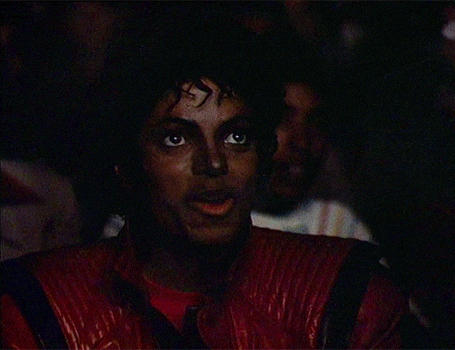General Topics
Markets
Technical Topics
Brokerage Firms
Company Specific
Community Lounge
Site Support

# Unique multifractal analysis

Discussion in 'Technical Analysis' started by Collapse, Aug 19, 2019.

1. Financial market – interdisciplinary formation. Ideas from different disciplines:
• fractal geometry
• chaos theory
• theory of self-organization (synergetics)
• economics
• physics
• functional analysis
• group theory
• heuristics
• ...
could be combined together in order to build full market axiomatics and create fundamental solutions. Obsoletes:
• mathematical statistics
• efficient market hypothesis
• time series
• moving averages
• fractal dimension
• volumes
• discreteness of Fibonacci levels
• linear indicators
• ...
Attractors (from dynamic chaos):
• points (fractal begin/end - static/dynamic indicators)
• curves (price retracement level, price limit - dynamic indicators)
• shapes (fractal boundaries - static indicators)
pull and push the price. So it is possible to have statistical advantage in trading.

Fractal in theory (Mandelbrot set):

Fractal in practice (recent EUR/USD chart):Fractal – hierarchical self-similar structure (some sort of market rebalancing). All fractals have different shape but the same formula. They could be generated by each other (crossed, moving in the same direction) or separated/sequential (as a rule: moving in inverse direction). Any indexes/crosses have the same fundamental rules.

It is possible to determine fractal end without delay. All algorithms require starting point as the main (and almost single) parameter (so there is no need to guess).

Chaos theory makes no predictions. Multifractal expansion forecast could be performed by the combination of group theory and quantum mechanics.

Last edited: Aug 19, 2019
2. Last edited: Aug 19, 2019
speedo and Fonz like this.
3. mr collapse,
Could you run a multifractal analysis on a 10m eur-201908-globex contract when you get a chance for time relevent?
thx

4. You will ultimately find that the simpler the better.

murray t turtle likes this.
5. tommcginnis likes this.
6. OP, you forgot one...

http://noosphere.princeton.edu/

Work all that into your data.Happy trails!

tommcginnis likes this.
7.8. Charts contain millions of fractals. It makes sense to calculate all of them only for HFT.

For the time being I'm performing analysis manually. And that requires some effort.

9. Recent EUR/USD chart (bifurcation point):Price limit (dynamic indicators):